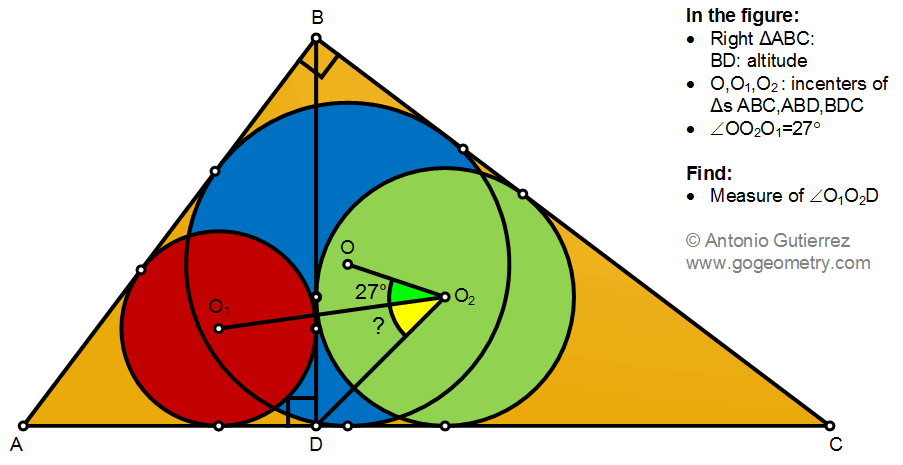# Geometry Problem 1492: Right Triangle, Altitude, Incenters, Angle, Measurement

The figure shows a right triangle ABC with altitude BD. Points O, O1, and O2, are the incenters of triangles ABC,ABD, and BDC. If the measure of angle OO2O1 is 27 degrees, find the measure of angle O1O2D.#### Incircle, Incenter

Incircle is a circle within a triangle, that is tangent to each side. The incenter is the center of the triangle's incircle.